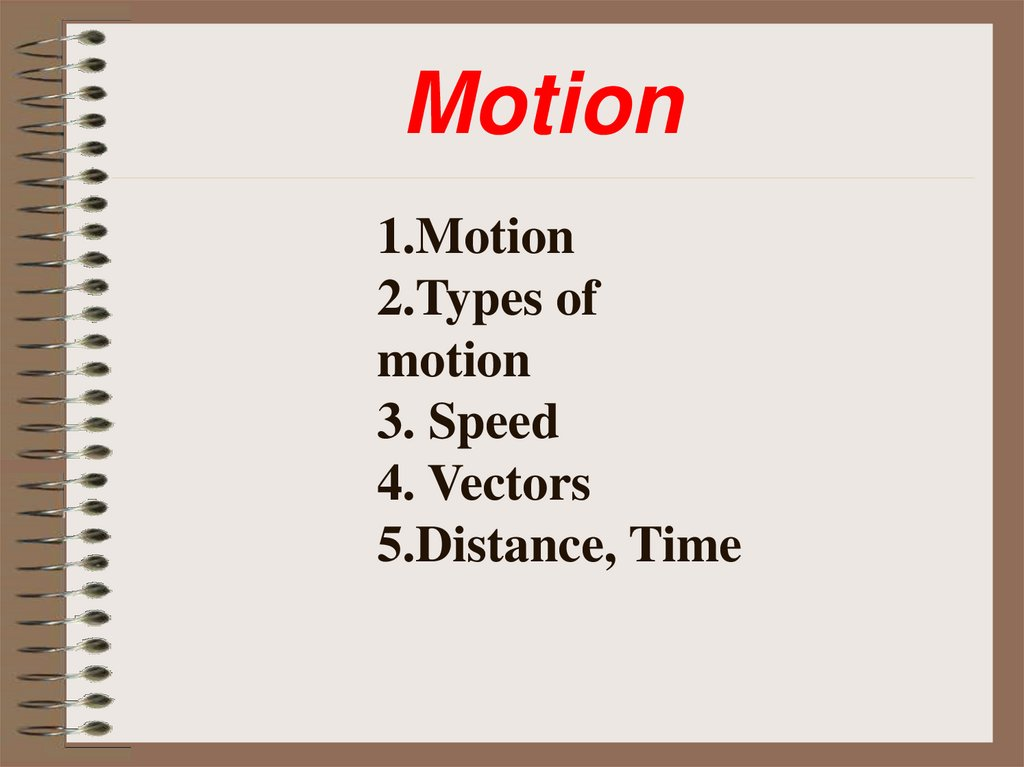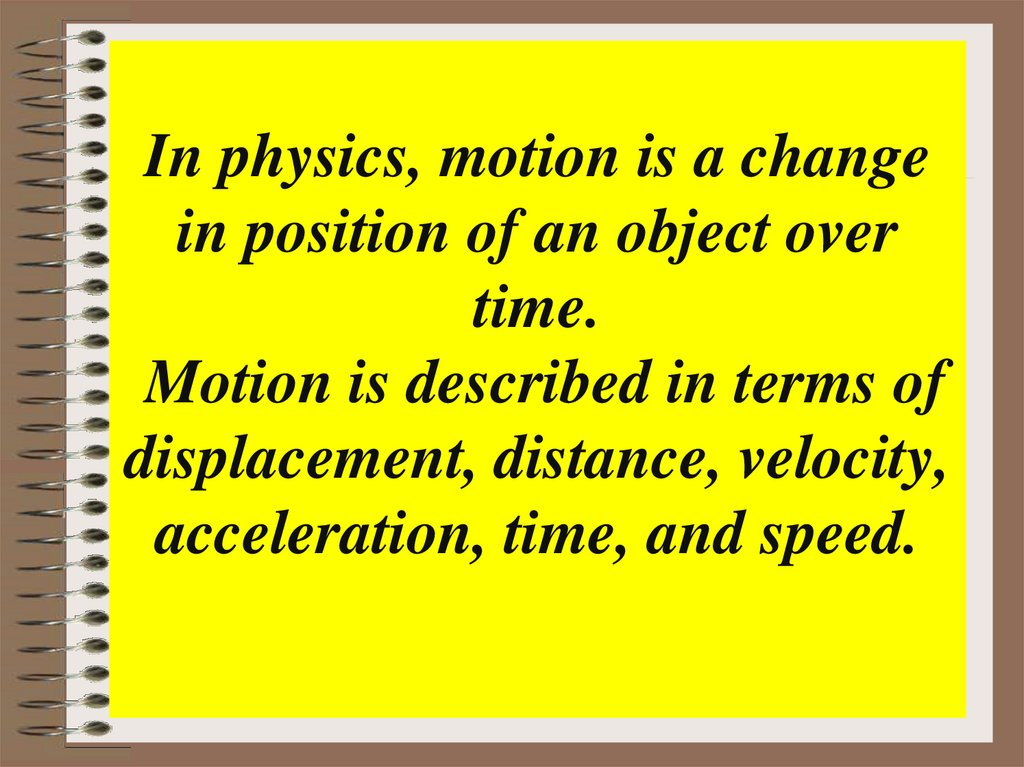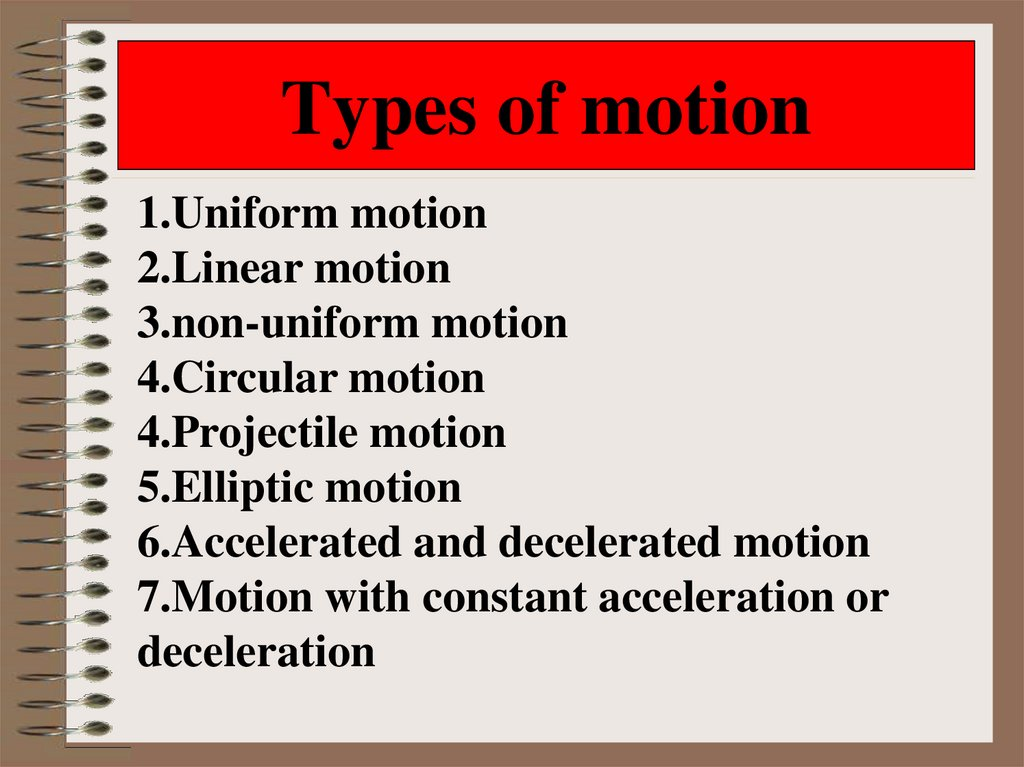# Motion. Types of motion

Motion
1.Motion
2.Types of
motion
3. Speed
4. Vectors
5.Distance, Time

## 2. In physics, motion is a change in position of an object over time. Motion is described in terms of displacement, distance,

velocity,
acceleration, time, and speed.

## 3. Types of motion

1.Uniform motion
2.Linear motion
3.non-uniform motion
4.Circular motion
4.Projectile motion
5.Elliptic motion
6.Accelerated and decelerated motion
7.Motion with constant acceleration or
deceleration

## 4.

If the body moves equally along
the same length, the motion is
called a uniform motion.

## 5.

If the body moves in an equally distinct time, it is
called non-uniform motion.

## 6.

What kind of motions do these photos refer
to?

Circular Motion

## 11.

2-1. Speed
• Definitions:
– Speed
• The rate at which something moves a given distance.
• Faster speeds = greater distances
– General formula for speed:
• Speed = distance / time
• Abbreviations commonly used:
d = distance t = time v = speed
v = d/t

2-1. Speed
Velocity
miles
d 100miles
v
40mph
40
hour
t 2.5hours
Distance
d v t 30 miles
6
hours 180miles
hour
Time
miles
d 100miles
t
2.5hours
2.5
miles / hour
v 40miles / hour

## 13.

2-1. Speed
Average speed is the total
distance traveled by an
object divided by the
time taken to travel that
distance.
Instantaneous speed is
an object's speed at a
given instant of time.

## 14.

2-2. Vectors
Magnitude of a quantity
tells how large the
quantity is.
Scalar quantities have
magnitude only.
Vector quantities have
both magnitude and
direction.

## 15.

2-2. Vectors
Velocity is a vector quantity that includes both speed and
direction.

## 16.

2- 4. Distance, Time
Vavg =
(V1 + V2)
(20mph + 60mph)
2
2
d = vavg t
= 40mph
30mph 2hr = 60miles

## 17. Semantic card

Uniform
motion
Oрын
Displasem
ent
Reference
point
Бірқалы
пты
қозғалыс
Tежелу
Uniform
motion
Үдеу
Decelera Position
tion
Санақ
нүктесі
Орын
Равноме ускорен
ауыстыр рное
ие
у
движени
е